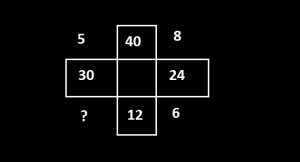Home > Math Shortcuts > Brain Trigger #19 – Sample Number puzzle question

# Brain Trigger #19 – Sample Number puzzle question

Almost every competitive exams have Number puzzle quetions. Here we will discuss few of them. Questions like Number puzzle will help you to prepare for your competitive exams. Try to Solve these questions with your math skills. You can also apply your learning skills of Shortcut Tricks.

Every types of Number puzzle problems and its shortcut tricks, how to do methods, you will find here in this website. what you need to do is to apply these tricks in Number puzzle problem to solve. You can solve these questions without using any shortcut tricks also.

Now try to solve a question on Number puzzle. All you need to do is to read the question very carefully and try to solve it by yourself. Answer of this question will be provided below. If you solve the question then check your answer with our solution. If you don’t know how to solve this then also check below.

A page contain a problem on Number puzzle and also its solution with explanation. By cliking on Next/Previous link you can navigate through other questions.

Let’s starts the question and answer session. Today’s question is:

what should be the number replaces the question mark ?Scroll down below to know the solution of this problem. You can take as much time as you need to solve the problem. But try to solve this as quicker as you can.

(Scroll down to get the answer)

(Scroll down to get the answer)

(Scroll down to get the answer)

(Scroll down to get the answer)

Here is the Answer of above question. If you already try the problem and got an solution then check your answer with our solution. Well Done if you correctly answer the previous question, but don’t worry if you are unable to answer the previous question correctly. Here is the answer of the question with explanation.

You can solve this Number puzzles in this way…….

40 – 30 = 10 / 2 = 5
40 – 24 = 16 / 2 = 8
24 – 12 = 12 / 2 = 6
30 – 12 = 18 / 2 = 9

 << PREVIOUS QUESTION NEXT QUESTION >>

Questions on some diferrent topics is also provided here. Click on Next/Previous link, it will take you to different topics.

If you have any question regarding this topic then please do comment on below section.You can also send us message on facebook.

1.